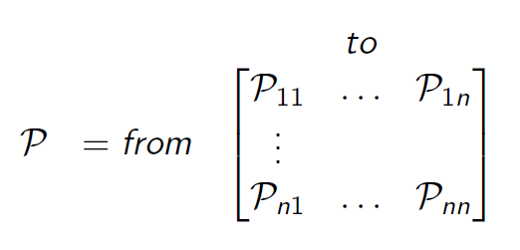Time-Series Analysis

By Prof. Seungchul Lee
http://iai.postech.ac.kr/
Industrial AI Lab at POSTECH

# 1. So Far¶

• Regression, Classification, Dimension Reduction,

• Based on snapshot-type data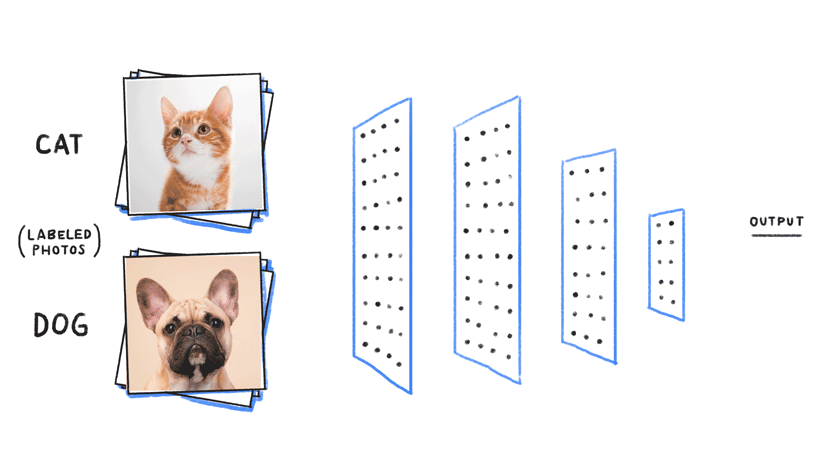• Sequence matters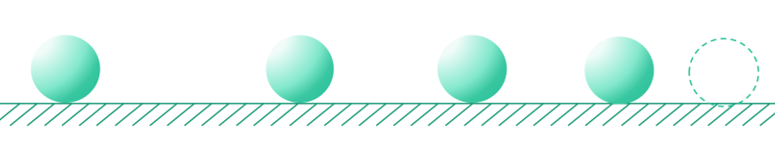• What is a sequence?
• sentence
• medical signals
• speech waveform
• vibration measurement
• Sequence Modeling
• Most of the real-world data is time-series
• There are important bits to be considered
• Past events
• Relationship between events
• Causality
• Credit assignment
• Learning the structure and hierarchy
• Use the past and present observations to predict the future

# 2. (Determinstic) Sequences and Difference Equations¶

We will focus on linear difference equations (LDE), a surprisingly rich topic both theoretically and practivally.

For example,

$$y=1,\quad y=\frac{1}{2},\quad y=\frac{1}{4},\quad \cdots$$

or by closed-form expression,

$$y[n]=\left(\frac{1}{2}\right)^n,\quad n≥0$$

or with a difference equation and an initial condition,

$$y[n]=\frac{1}{2}y[n−1],\quad y=1$$

High order homogeneous LDE

$$y[n]=\alpha_1 y[n−1] + \alpha_2 y [n−2] + \cdots + \alpha_k y[n-k]$$

# 3. (Stochastic) Time Series Analysis¶

## 3.1. Stationarity and Non-Stationary Series¶

• A series is stationary if there is no systematic change in mean and variance over time

• A series is non-stationary if mean and variance change over time

• Example: GDP, population, weather, etc.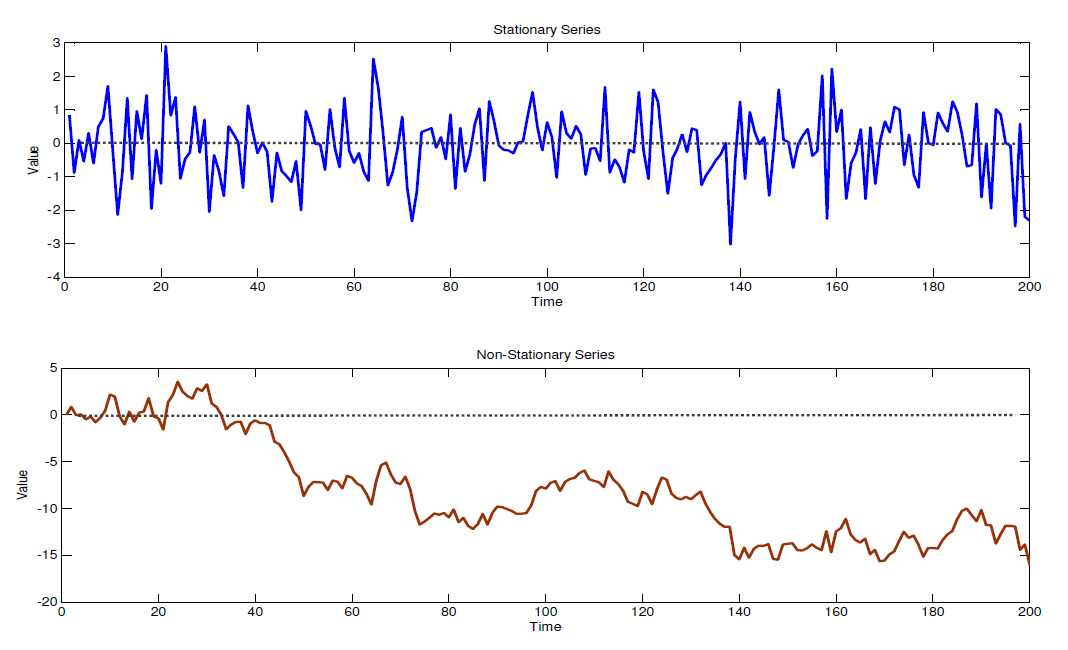## 3.2. Testing for Non-Stationarity¶

Formally

• Augmented DickeyFuller test

Informally

• Auto-Correlation Function (ACF)
• Normal Quantile Plot (Q-Q plot)

Q-Q Plot

• Compare distribution of the residuals to normal
• Scatter plot of residual quantiles against normal
• Stationary data: quantiles match normal ($45^o$ line)
• Non-stationary data: quantiles do not match (points off $45^o$ line)

## 3.3. Dealing with Non-Stationarity¶

Linear trends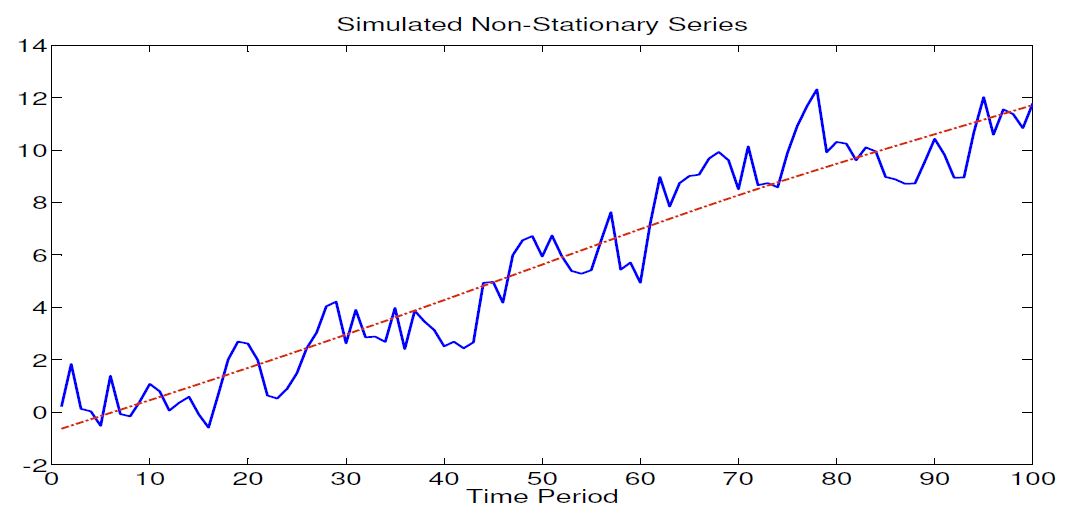Non-linear trends

• For example, population may grow exponentially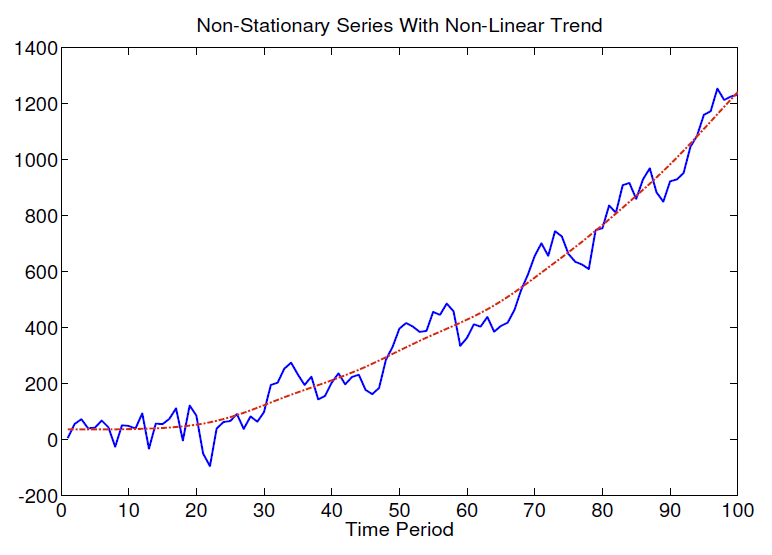Seasonal trends

• Some series may exhibit seasonal trends

• For example, weather pattern, employment, inflation, etc.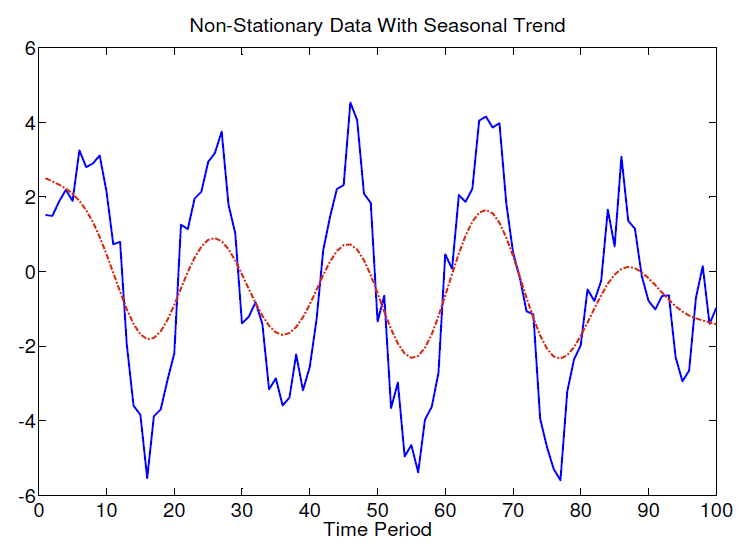Combining Linear, Quadratic, and Seasonal Trends

• Some data may have a combintation of trends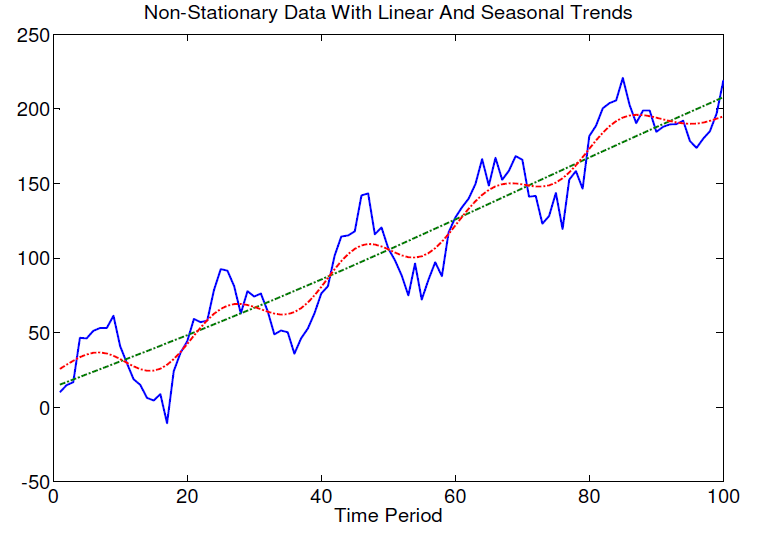• One solution is to apply repeated differencing to the series

• For example, first remove seasonal trend. Then remove linear trend

• Inspect model fit by examining residuals Q-Q plot

• Anternatively, include both linear and cyclical trend terms into the model
\begin{align*} Y_t &= \beta_1 + \beta_2 Y_{t-1} \\ &+ \beta_3 t + \beta_4 t^{\beta_5} \\ &+ \beta_6 \sin \frac{2\pi}{s}t + \beta_7 \cos \frac{2\pi}{s}t \\ &+ u_t \end{align*}

# 4. Markov Process¶

## 4.1. Sequential Processes¶

• Most classifiers ignored the sequential aspects of data
• Consider a system which can occupy one of $N$ discrete states or categories $q_t \in \{S_1,S_2,\cdots,S_N\}$
• We are interested in stochastic systems, in which state evolution is random
• Any joint distribution can be factored into a series of conditional distributions

$$p(q_0,q_1,\cdots,q_T ) = p(q_0) \; p(q_1 \mid q_0) \; p( q_2 \mid q_1 q_0 ) \; p( q_3 \mid q_2 q_1 q_0 ) \cdots$$

Amost impossible to compute !!

$$p(q_0,q_1,\cdots,q_T ) = p(q_0) \; p(q_1 \mid q_0) \; p( q_2 \mid q_1 ) \; p( q_3 \mid q_2 ) \cdots$$

Possible and tractable !!

## 4.2. Markov Process¶

• (Assumption) for a Markov process, the next state depends only on the current state:

$$p(q_{t+1} \mid q_t,\cdots,q_0) = p(q_{t+1} \mid q_t)$$
• More clearly

$$p(q_{t+1} = s_j \mid q_t = s_i) = p(q_{t+1} = s_j \mid q_t = s_i,\; \text{any earlier history})$$

• Given current state, the past does not matter
• The state captures all relevant information from the history
• The state is a sufficient statistic of the future

## 4.3. State Transition Matrix¶

For a Markov state 𝑠 and successor state 𝑠′, the state transition probability is defined by

$$P_{ss'} = P\left[S_{t+1} = s' \mid S_t = s \right]$$

State transition matrix $P$ defines transition probabilities from all states $s$ to all successor states $s'$.